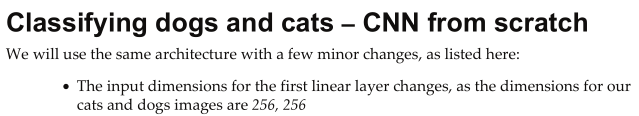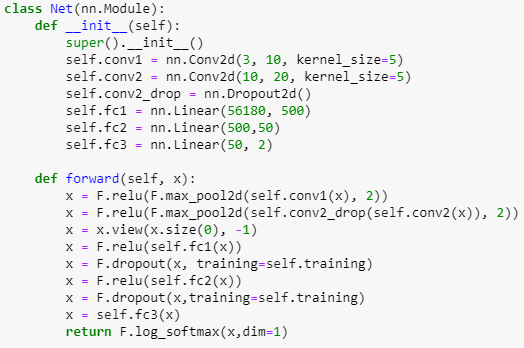# Binary classifier Cats & Dogs questions

Hi all, I’m currently looking at Pytorch tutorials online & going thru a PacktPublishing book called “Deep Learning with PyTorch” by Vishnu Subramanian and I had some questions I hope some of the more experienced ML/data science comrades could help me with.

1)The book stated the cat and dog images were 256x256but it dosn’t make sense to me because later on the line of code was used:

simple_transform = transforms.Compose([transforms.Resize((224,224))
,transforms.ToTensor()
,transforms.Normalize([0.485, 0.456, 0.406], [0.229, 0.224, 0.225])])

If the images were rescaled to 224x224, then why say there were 256x256? I checked the dogs-vs-cats dataset from kaggle in the folder and all the images are of different a various sizes much bigger or smaller than 256x256 (essentially all are randomly sized).

1. This is a screen shot of the Neural Network class I used for the Cat & Dog binary classifier CNN:If the input 3 channel RGB cat/dog images are 224x224, I understand how Convo2d with a 5x5 kernel outputs 10 @ 220x220 and then max_pool2d with a 2x2 kernel to 10 @ 110x110, then Convo2d again to a 10 @ 106x106 and then max_pool2d to 20 channels of 53x53 size which equals 56180, which is the input to the first nn.Linear layer (self.fc1) in my network, BUT how or why is the output of the first linear layer calculated to be 500? i.e. self.fc1 = nn.Linear(56180, 500) ? furthermore, for the ouput of the second linear layer 50? (I know 2 is the output of the third/last linear layer because its a binary classifier and either chooses dog or cat).

2. When downloading the dogs-vs-cats dataset from kaggle, https://www.kaggle.com/c/dogs-vs-cats/data it seems the “train” folder which held 25000 images of cats and dogs inside of it was used to create a “validation” dataset the book called it.

I used these lines of code to , which created a new “valid” folder that didn’t exist inside the main folder dogs-vs-cats (which held the “train” folder that had the 25,000 images).

Then, the lines of code were used to create “cat” and “dog” sub-folders in BOTH “train” and “valid” folders inside the “dogs-vs-cats” folder that held them both:

I was looking at the folder as I ran the lines of code above in Jupyter notebook and could see the files getting copied when I ran the first for loop i.e. "for i in shuffle[ : 2000 ]: " I had to use shutil.copy instead of shutil.copyfile that they used in the book because I was getting a permission error for reading and writing files ( I tried running Conda prompt as adminstrator and then launching Jupyter notebook off it to no avail).

I saw that the 2,000 unique images of cats and dogs were MOVED (not copied) from the “train” folder which meant 25,000 - 2,000 = 23,000. So now the “train” folder held 23,000 images BUT now the “valid” folder held 4,000 images because it looked like it moved the cat and dogs images to the main “valid” folder and then COPIED them again to the “cat” and “dog” subfolders within which was weird to me (essentially creating duplicate images). Is this correct? Could it be because I used shutil.copy instead of shutil.copyfile?:

After running the second copy for loop i.e. "for i in shuffle[2000: ]: ", it seemed like the remaining 23,000 images in the “train” folder where placed in their corresponding “cat” and “dog” subfolders, but also COPIED over the main “valid” folder, which already held 2,000 images and not totaled 25,000 once again (i.e. 23,000 + 2,000). So then “train” folder looked like this after:

I was confused why the images were getting swapped over like that? Or I feel like some of these images are getting COPIED when they should be simply getting moved, because I essentially have a duplicate images files in my “valid” folder now.

Also, how does one go about selecting the batch size? I used 32 here per the tutorial, but is it totally arbitrary? and the For loops for the epoch training? Does the amount of loops through your data depend on the batch size or amount of images you have?

Sorry about all the questions and hopefully I was not confusing but I would appreciate any help provided.

1: If you resize the image using transforms.Resize(244,244) all images will be of the size 244 so if the book says the input to the first layer is 256 it is wrong it will be [batch_size, 3,244, 244]
If you use the Kaggle dataset (I found out first hand) you should resize them so they are all the same size or you will get errors.
you can do this if you are not 100% sure about the shape of the tensor when running the code; in your Forward method do this

``````   def forward(self, x):
print(x.shape)
x = self.pool(torch.relu(self.conv1(x)))
x = self.pool(torch.relu(self.conv2(x)))
x = self.pool(torch.relu(self.conv3(x)))
print(x.shape)
# Flatten image
x = x.view(x.size(0), -1)
x = self.dropout(x)
x = torch.relu(self.fc1(x))
x = self.dropout(x)
x = self.fc2(x)
return x
``````

So using the print(x.shape) will give you the tensors shape so you are 100% sure what is going in and out of the model.

For 2: This I think is called downsampling so you are making the data smaller by removing what is not ‘needed’ or ‘noise’ in the image. I have seen them go both down and up in size in the FC layers.

3: For this what you should have in your dataset is two main folders a train and a validation folder. These two folders will then have both a cat and a dog folder.
So the structure would be like this
dataset/train/
…/cat/
… /dog/

dataset/valid/
…/cat/
… /dog/
Also, there should be no duplicate images in the folders. The code does not look like it is checking if the image exists or not. But you are right when thinking that the images should be moved rather than duplicated/copied. You do not want to have the same image in the train/valid/test folders as this will cause a sample bias in your dataset. So what you have looked ok but you will have to add the train path carefully like this
path = …/train/train/dog/

I hope that helps out a bit.

Oh wow I realized that this is a year old post.